Home

# Electric Fields

Much has been said in the preceding chapters about something called the electric field and how it is responsible for things like electrical and magnetic forces.  So what actually is an electric field?

Putting aside equations, the general definition is along the lines: “a region of space surrounding a charge where another charge experiences a force”.

Stated like this, the field sounds like an abstract notion, almost like a mathematical concept that serves no purpose other than to enable one to calculate forces.  Or perhaps it describes an altered property of empty space.  If so, this would appear a contradiction since empty space should have no properties.

#### Static or flowing?

Is an electric field something that stands still or something that flows?  In general, a field that doesn’t change its intensity is described as ‘static’.  A field that fluctuates in intensity might be described as ‘dynamic’, but not flowing as such.  Is that an accurate description?

We know that the effects of an electric field propagate at a finite speed – that of light.  If we could suddenly introduce an identical charge nearby another, the existing charge would not respond immediately.  Instead there would be a brief pause before the existing charge began accelerating away.  This would seem to indicate there is something physically moving from one charge to the other and then striking that second charge with a force.

But then again, a scientist might argue that there is nothing moving between the charges.  Instead, what we observe it is just the effects of ‘relativity of simultaneity’.  By this he means, it takes a while for the presence of a charged particle in one location to become true at another, and this delay in presence is determined by distance and the speed of light.

Such explanations can be confusing and quite contrary to our common sense.  As a scientific principle, it is always best to consider logical explanations before turning elsewhere.  By ‘logical’ we mean something that can be modeled and understood in terms of other processes, particularly those at a larger scale.

Let’s take a river as an example.  Is a river static or flowing?

If we view a river from high above, all we see is a fixed body of water.  Just a line on a map and we would probably describe it as static.  When viewed from ground-level, the picture is not much different.  If the water contains no debris and no turbulence, the river gives no indication of its underlying nature and might still be described as static.

If we throw something into the river like a leaf and see it float downstream, at that point we might suspect the river itself is also moving with the leaf.  But then again, a scientist might argue “No, the river is standing still.  The leaf moves because the water applies a force to it.”

But of course no scientist would seriously say that.  We know perfectly well that the river is not static at all.  The water is flowing and that is what pushes the leaf along.

Could an electric field be a similar phenomenon?  Could what we describe as an electric field be in fact, not just be an abstract mathematical entity, but an actual flow of material that moves outward from a charge and imparts a force on other charges when it hits them?

On one hand this sounds logical because it explains why there would a delayed effect if the ‘material’ moved at the speed of light.  It would also explain how an electric force is exerted at a distance:  it isn’t, the force is applied directly when the material meets/hits the other charge.

On the other hand it sounds illogical because the material is not coming from anywhere.  It flows out of the particle, yes, but without first coming into it from somewhere else.

So what if the electric field ‘material’ was not coming from elsewhere but being generated ‘on the fly’?  That is, it was continuously being produced and then ejected?

This would seem impossible because it violates conservation laws.  As we know, quantities like mass and charge are conserved and can’t just be created anew.  At least not without being created in neutral positive/negative pairs (in the case of particles) or converted from other forms (in the case of mass).

But note that the electric field material described here contains no mass or charge.  So a continual creation of this substance, whatever is was, would not violate any known conservation laws.  The only thing it would do is apply a continual force on another charge standing in its path – something we observe as true.  Therefore the process could continue forever without increasing the amount of ‘normal matter’ (subatomic particles) in the universe.

For the sake of description let’s call this material ‘field substance’ and state the above as a hypothesis.

The Electric Field Hypotheses:

Each charged particle generates something called ‘field substance’ which makes up what we call an electric field.  This substance is continuously and perpetually generated within charged particles and moves away at the speed of light (relative to the particle).  As it spreads spherically outward it becomes thinner and thinner.  This substance is a not a mathematical abstraction, but an actual physical substance that travels through space.  When it strikes another charged particle it exerts a force.  The amount of force and its direction will be based on charge values, local field substance density, and relative velocities.

#### Analogies

In order to visualize what is being described it is best to make some analogies.  There are a number of ways of thinking about this.  One is to imagine a series of rubber balloons that start at the surface of a (spherical) charged particle and expand outwards.  As they do, they become more and more stretched, but never burst.

Another way is to a imagine a fine mist of particles being sprayed outward.  As they do, their density becomes less and less dense, which means fewer will hit a target further away and thus exert less force.

These animations should explain what is meant.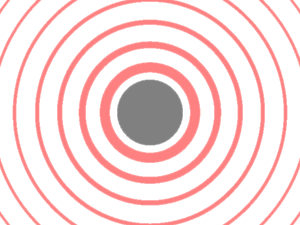Left: a series of rubber balloons, representing ‘field substance’, form at the surface of a charged particle and expand outwards, becoming increasingly thinner but without ever bursting.
Right: tiny particles are being generated within a charge and ejected at the speed of light.

These are just analogies, mind.  Perhaps it would be more accurate to describe a continuous, infinitely-stretchable lump of rubber.  But that is difficult to draw.

For various reasons, the second method of imagining the field to be made of tiny particles will be the most convenient model to work with.  That doesn’t mean it is correct, just that it is the simplest way of imagining the interaction between field and charge.  In this way we can consider the particles as ballistic projectiles that exert a force on a target.  Another thing that should be obvious from this is that the density of particles will decrease with inverse square of distance, thus bringing their impact force in line with the Coulomb force.  This is because they are evenly spread over the surface a sphere whose area increases with distance squared.

Perhaps the simplest direct analogy for this ballistic particle model would be the solar wind coming from the Sun, and how the wind exerts a force on objects such as ‘solar sails’ (which are not reality as yet).

#### Field lines

Quite often electric fields are described and drawn in terms of lines that come in and out of, and curve around, charged particles.  While such drawings can be useful, it is important to remember that they are only concepts for showing force directions and don’t necessarily represent anything physical.   Unfortunately this has led to major misconceptions in our understanding of fields.

Consider this electric field diagram: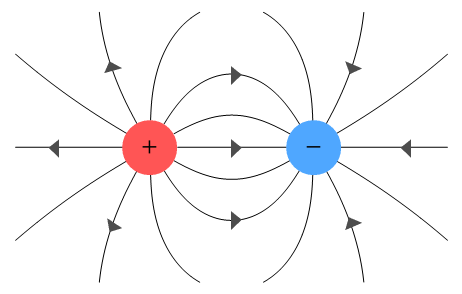This oft-cited presentation shows a positive and negative charge. The field starts at the positive charge and moves outward. There are no charges on its left. So the field moves away in mostly straight lines (with the left-most line being exactly straight) and steadily weakens with distance. To the right is a negative charge. So the field bends its way toward that charge and disappears into it. Meanwhile there is nothing to the right of the negative charge, so a field seems to appear from nowhere and move toward that charge.

This concept is surely wrong. An electric field could not bend toward another charge because the field doesn’t know where that charge is before it gets there. When we walk we can see ahead and use that information to decide our direction because our sight is much faster than our legs. But an electric field is already moving at light speed and cannot ‘see ahead’ any faster than it is moving. Additionally, the field should be shown running in both directions because the positive-negative distinction is arbitrary.

A more accurate version of events would be in this diagram: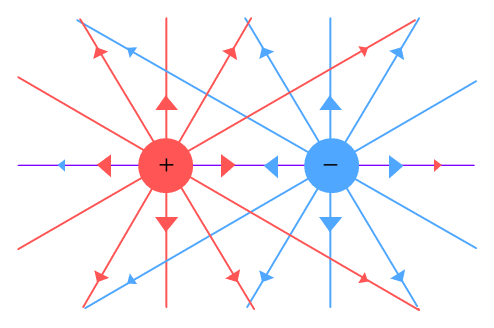Here we see both charges generating a field, and both fields extending directly away from their charges, in straight lines, and weakening with distance. The previous diagram may be accurate if it were describing the net force experienced by a nearby separate positive charge, but not as a general field-line diagram.

#### Modelling the forces

When electric field strikes a charged particle it exerts a force.  On an intuitive level this makes sense.  If we were to spray a material substance, such as a jet of air, at an object we would exert a force on that object and it would be pushed away.

But with charged particles the direction of force is not always intuitive. There are two types of interactions we are dealing with: the repulsive force between like charges; and the attractive force between opposite charges.  In trying to understand how things work at a ‘too-small-to-see’ microscopic level, it is useful to make comparisons with things at an ‘everyday-world’ macroscopic level.

For the repulsive force this is easy.  Imagine a stream of tiny bullets emerging from a charged particle.  These bullets emerge from all directions and travel directly away from the particle at light speed.  A similarly charged particle nearby would feel the force of these bullets and be repelled by them.  The closer the second particle was to the first, the more bullets would hit it, and hence the force would be greater.  If the particle was further away, fewer bullets would hit and the force would be less.

In keeping with the Velocity Dependent Coulomb’s Law (VDCL) discussed earlier, as the particles moved inward, the impact speed of the bullets, and hence the force, would increase.  As the particles moved outward this would lower the impact speed and force.  And if that second particle moved away from the first at the same speed as the bullets (i.e. at light speed), then the bullets would not hit and hence could apply no force at all.

So what analogy could we make for the attractive force?  Again this looks easy.  Simply reverse the direction of the bullets so that they dive inward toward the charged particle.  Unfortunately there are major problems with that idea.  In order for the bullets to move toward the particle they would have to know in advance, not only the position of the particle, but also its velocity.  They need to know the particle’s velocity because the bullets must strike that particle at light speed.  The bullets would also need to emerge at the beginning of time, from the furthest reaches of the universe, and somehow affect other particles in reverse order – i.e. impact particles closest to their destination first.  This involves a level of clairvoyance and inverse time travel far beyond what any sane scientist would consider feasible.  Obviously this model is wrong and we need a different approach.

#### A new model of electric forces

In order to explain the interaction between of oppositely charged particles, we can look at electric fields and forces as follows:

Each charged particle emits a field type corresponding to its charge.  Positively charged particles emit a ‘positive field’ and negatively charged particles emit a ‘negative field’.  The field itself however contains no charge, just information about the charge it originated from.  So we can describe the field as having a ‘sign’, i.e. positive or negative.

These fields emerge from all ‘sides’ of the particle and move directly outward at exactly light speed relative to their starting point.  When they meet another particle one of the fields will interact with it, either pushing or pulling, depending on that particle’s charge.

How do these fields interact?  We already have a simple analogy for the pushing process, so let’s now look at the pulling process.   The field emanates from the charged particle and moves away from it at light speed.  When it hits an oppositely charged particle, the field can be considered as making a sudden ‘U-turn’ and pushing that particle in the opposite direction, i.e. pulling it.  The pulling action happens at light speed and that particle receives the same (but opposite) force as a like-charged particle would from the same field.  This diagram represents the action: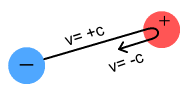For an analogy, consider a paddle steamer.  As the big boat steams along the river, its paddles reach out and pull the water in the opposite direction, much like a swimmer’s hands.  Now suppose this old-style boat had been equipped with a modern, stronger propeller engine that was able to move the boat in either direction.

If the propeller were to pull the boat backward, the force of the paddles on the water would increase.  If the propeller were to gently push forward, the force of the paddles on the water would decrease.   If the propeller pushed at the same speed as the paddles, the paddles would exert no force on the water.  And if the propeller pushed even faster, the paddles would now begin to push against the water.

Okay so there are no bullets or paddle steamers at the atomic level.  These are just models to help us visualize what’s going on.  However there is a separate point to this; especially the pull-field U-turn model, which will be made use of in the next section.

#### Field Velocity

Time to introduce a new concept: Field Velocity. When charged particles interact it is not the particles themselves that pull or push on each another, rather it is their fields. The Velocity Dependent Coulombs Law (VDCL) tells us to look at each (source) particle’s velocity and then, depending on the charges, add or subtract this velocity to the speed of light to determine how much impact it will have on another (target) particle. This net velocity is then cubed to determine the degree of force applied.

Since the target particle only interacts with a source particle’s field however, it makes more sense to talk only about this net velocity of the field. This velocity, herein called the Field Velocity, is defined as the velocity at which an electric field strikes a target particle. In a situation where a source particle is moving at velocity v toward a target (see below diagram), the Field Velocity vf will be:

vf = c + v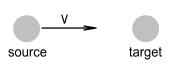This new velocity vf will be greater than light speed c if the source is moving toward the target, and less than c if moving away from the target. Importantly, this velocity vf is defined the same way for both like and oppositely charged particles.

#### Using Field Velocity in the VDCL

Now that we have defined Field Velocity we can use it in the VDCL equation. But instead of having a single equation we’ll have a separate one each for ‘push fields’ and ‘pull fields’. Here they are:

VDCL for Push fields: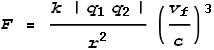VDCL for Pull fields:Here are some points to note:

1. When the particle velocities are zero, i.e. at rest relative to each other, both equations become the same and equal to Coulomb’s Law.

2. The signs on the charges are not important in the equations because we choose which force equation to use based on the charge signs. Thus only the absolute values of the charges appear in the equations (between the “|” symbols).

3. For both equations, a positive result means repulsion and a negative result means attraction. Hence it is possible for a ‘push field’ to pull and vice-versa. Although this will only happen for particle velocities above light speed.

4. The only difference between Push and Pull is that Pull contains a -2c term. This represents the U-turn made by a ‘pull field’ when it meets a target particle: the field reverses its direction at double the speed of light relative to its incoming speed. This is the same thing as you would have in a car U-turn: in order to be eventually moving backwards at the same as your forward speed, your net change in velocity must be the negative of double your incoming velocity.

You may be wondering what the point is in having separate equations (and making things more complicated!). The reason is partly because it better represents the field models above. But a more important reason for this will become clear in a later chapter about ‘sideways forces’.

#### A correct representation of magnetic fields

Some final points before moving ahead. I wish to a correct a common misrepresentation of magnetic field lines. Magnetic fields are often drawn as circling a wire. A diagram of such looks like this:In this diagram the magnetic field lines seem to start at various distances from the wire and then proceed to circle it at fixed radii. There is also usually a picture (not included) of a hand beside the diagram showing a ‘thumbs up’ to tell you about the right-hand rule. The implication of this diagram is that magnetic force acts at right angles and would force something to circle the wire.

But this is clearly untrue. When two current carrying wires are placed in parallel, the only force between them is either directly toward or directly away from each other. The wires are never pushed sideways, as if to orbit each other as the diagram implies; nor could they be. If a force is caused by a wire then that force must come from that wire. In other words we wouldn’t expect a force to appear at a certain distance away and then travel sideways. Here is a more realistic representation showing the force lines moving outward: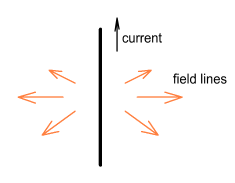Similarly, the force lines from a coil of wire are also misrepresented. They show the field lines emerging from the centre of the coil, when there is nothing at that centre for the field to emerge from. Again the actual field must come from the coils itself. Here is a before and after correction diagram:The net force may feel like it’s coming from the centre, but it’s really coming from the wire all around it. A simple experiment involving an electric coil hovering over a paperclip shows the clip being attracted to the edge of the coil and not its centre. In fact, for a large diameter coil, the force at its centre is particularly weak.

#### Conclusions

Electric fields are commonly described in an abstract manner that does not explain how or why charges interact at a distance.  But by considering the electric field as physical substance we can describe a model for how the electric force takes place, why it varies with inverse square of distance, why its effect is delayed, and why the force appears to vary as a function of relative velocity.

 Previous Contents Next chapter